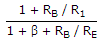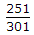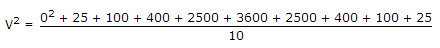Electronics and Communication Engineering - Electronic Devices and Circuits

41.

Calculate the stability factor and change in IC from 25°C to 100°C for, β = 50, RB/ RE = 250, ΔIC0 = 19.9 nA for emitter bias configuration.

 A. 42.53, 0.85 μA B. 40.91, 0.58 μA C. 40.91, 0.58 μA D. 41.10, 0.39 μA

Explanation:

SICO = (1 + β).51.= 42.53

ΔIC = (SICO).ΔICO

= 42.53 x 19.9 nA

= 0.85 μA.

42.

A periodic voltage has following value for equal time intervals changing suddenly from one value to next... 0, 5, 10, 20, 50, 60, 50, 20, 10, 5, 0, -5, -10 etc. Then rms value of the waveform is

 A. 31 V B. 32 V C. insufficient data D. none of these

Explanation:RMS value = 965 = 31.064 Volt.

43.

Work function of oxide coated cathode is much lower than that of tungsten cathode.

 A. True B. False

Explanation:

Therefore emission current from oxide coated cathode is more.

44.

The word enhancement mode is associated with

 A. tunnel diode B. MOSFET C. JFET D. photo diode

Explanation:

MOSFET may be depletion mode or enhancement mode.

45.

In which region of a CE bipolar transistor is collector current almost constant?

 A. Saturation region B. Active region C. Breakdown region D. Both saturation and active region